# Localization at a submonoid

## Definition

Let$R$ be a commutative unital ring and$D$ be a submonoid of$R$ not containing zero (in other words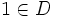$1 \in D$ and$D$ is closed under multiplication). Such a$D$ is also termed a multiplicatively closed subset. The localization of$R$ at$D$, denoted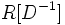$R[D^{-1}]$ or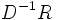$D^{-1}R$, is defined as follows:

• Set-theoretically, it is the set of ordered pairs$(a,b)$ with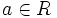$a \in R$ and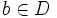$b \in D$ modulo the following equivalence relation: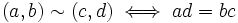$(a,b) \sim (c,d) \iff ad = bc$

The pair$(a,b)$ is denoted as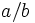$a/b$ \r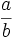$\frac{a}{b}$.$a$ is termed the numerator and$b$ the denominator of the fraction$a/b$.

• The operations are as follows: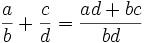$\frac{a}{b} + \frac{c}{d} = \frac{ad + bc}{bd}$

and: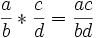$\frac{a}{b} * \frac{c}{d} = \frac{ac}{bd}$

(It needs to be checked that these operations go down under the equivalence).$D^{-1}R$ is a commutative unital ring and$R$ is the subring comprising those elements with denominator$1$.

## Related notions

• Localization at a prime ideal: The terminology is somewhat misleading, because this actually means localization with respect to the submonoid which is the set-theoretic complement of the prime ideal.
• Field of fractions: When the ring is an integral domain, we can choose the submonoid as the set of all nonzero elements (equivalently, we are localizing at the zero ideal). The ring obtained is a field, termed the field of fractions of the integral domain.
• Total quotient ring: Here, the submonoid is the set of all nonzerovisors. This generalizes the notion of field of fractions to rings that are not integral domains.